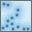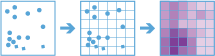Calculate DensityThe Calculate Density tool uses input point features to calculate a density map within an area of interest.

Workflow diagramAnalysis using GeoAnalytics Tools

Analysis using GeoAnalytics Tools is run using distributed processing across multiple ArcGIS GeoAnalytics Server machines and cores. GeoAnalytics Tools and standard feature analysis tools in ArcGIS Enterprise have different parameters and capabilities. To learn more about these differences, see Feature analysis tool differences.

Examples

• Bird counts can be used to calculate species densities. The densities can then be compared to land-cover data to determine which habitats each species prefers.
• The density of GPS measurements of flight paths can be calculated to determine which areas of airspace have high volumes of traffic.

Usage notes

The Calculate Density tool requires a single input of point features.

Density can optionally be calculated using one or more count fields. A count field is a numerical field that specifies the number of incidents at each location. Features such as cities or highways can use a count field when calculating the density of population or lanes of traffic, respectively. If you specify a count field, the density will be calculated for the count field in addition to the density of points.

Input points are aggregated into bins for analysis. You must specify the bin size to aggregate data into. Output results will be in square miles or square kilometers, depending on the Units setting in your profile. These defaults can be changed using the tool parameter Choose the output units for density drop-down menu.

You can provide the area layer to use for analysis, or you can generate bins of a specified size and shape (hexagon or square) to aggregate into. If you are aggregating into hexagons, the bin size d is the height of each hexagon, and the width of the resulting hexagon will be two times the height divided by the square root of three. If you are aggregating into squares, the bin size d is the height of the square, which is equal to the width.

You must specify a neighborhood size that is greater than the bin size. The neighborhood size is used to find input features within the same neighborhood as the feature (bin) of interest.

There are two options to calculate density: The Uniform option sums all the values within the neighborhood and divides them by the area. The Kernel option weights values in the neighborhood by distance from the feature of interest and applies a kernel function to fit a smooth tapered surface to each point.

Calculate Density allows you to optionally analyze using time stepping. Each time slice is analyzed independent of features outside of the time step. To use time stepping, your input data must be time enabled and represent an instant in time. When time stepping is applied, output features will be time intervals represented by the fields START_DATETIME and END_DATETIME.

Only areas within the neighborhood of a bin containing points will be returned.

Calculate Density requires that your area layer be in a projected coordinate system. If your data is not projected and you do not set a projected coordinate system, analysis will be completed in World Cylindrical Equal Area (wkid 54034).

If Use current map extent is checked, only the features that are visible within the current map extent will be analyzed. If it's not checked, all input features in the input layer will be analyzed, even if they are outside the current map extent.

Limitations

Density can be calculated for point features only.

ArcGIS API for Python example

The Calculate Density tool is available through ArcGIS API for Python.

This example calculates the density of crimes using 1-kilometer bins, a 2-kilometer neighborhood, and a 1-day time step.

# Import the required ArcGIS API for Python modules
import arcgis
from arcgis.gis import GIS
from arcgis.geoanalytics import analyze_patterns

# Connect to your ArcGIS Enterprise portal and check that GeoAnalytics is supported
portal = GIS("https://myportal.domain.com/portal", "gis_publisher", "my_password", verify_cert=False)
if not portal.geoanalytics.is_supported():
print("Quitting, GeoAnalytics is not supported")
exit(1)

# Find the feature layer to use for analysis and only use the features where a burglary occurred
crimes = portal.content.search("Crimes", "Feature Layer")
crimes_layer = layer_result.layers
crimes_layer.filter = "Burglary = 'TRUE'"

# Set the tool environment settings
arcgis.env.process_spatial_reference = 32618
arcgis.env.verbose = True

# Calculate crime density daily using 1-kilometer hexagon bins with a 2-kilometer radius
calculate_density_result = analyze_patterns.calculate_density(input_layer = crimes_layer,
weight = 'Uniform',
bin_type = 'Hexagon',
bin_size = 1,
bin_size_unit = "Kilometers",
time_step_interval = 1,
time_step_interval_unit = "Days",
output_name = "crime_density")

# Visualize the tool results if you are running Python in a Jupyter Notebook
processed_map = portal.map('NYC, NY', 10)
processed_map

Similar tools

Use Calculate Density to create a density map using point measurements. Other tools may be useful in solving similar but slightly different problems.

Map Viewer analysis tools

If you would like to calculate density on line features or using the standard analysis tools, use the Calculate Density tool.

If you would like to aggregate data without applying a neighborhood or weighting function, use the Aggregate Points tool.

If you want to find statistically significant clustering in point or area features, use the Find Hot Spots tool.

If you want to predict values based on point measurements, use the standard tool Interpolate Points.

ArcGIS Desktop analysis tools

The Calculate Density tool performs a similar function to the Kernel Density and Point Density tools.